Hu, W., and Liu, N. (2020). "Comparisons of finite element models used to predict bending strength of mortise-and-tenon joints," BioRes. 15(3), 5801-5811.

#### Abstract

This study aimed to obtain a better method for establishing a finite element model of mortise-and-tenon (M-T) joints. Three types of M-T joint finite element models, which included a whole rigid model, a tie rigid model, and a semi-rigid model, were established and compared with experimental results by predicting the bending moment capacity (BMC) of M-T joints based on the finite element method (FEM). The results showed that the semi-rigid model performed much better than the tie rigid model, followed by the whole rigid model. For the semi-rigid model, the ratios of FEM ranged from 0.85 to 1.09. For the whole rigid model and tie rigid model, the BMC of the M-T joint was overestimated. In addition, the results showed that tenon size remarkably affected the BMC and stiffness of the M-T joint, and tenon width had a greater effect on the BMC of the M-T joint than the tenon length.

Comparisons of Finite Element Models Used to Predict Bending Strength of Mortise-and-tenon Joints

Wengang Hu a,b,* and Na Liu b

This study aimed to obtain a better method for establishing a finite element model of mortise-and-tenon (M-T) joints. Three types of M-T joint finite element models, which included a whole rigid model, a tie rigid model, and a semi-rigid model, were established and compared with experimental results by predicting the bending moment capacity (BMC) of M-T joints based on the finite element method (FEM). The results showed that the semi-rigid model performed much better than the tie rigid model, followed by the whole rigid model. For the semi-rigid model, the ratios of FEM ranged from 0.85 to 1.09. For the whole rigid model and tie rigid model, the BMC of the M-T joint was overestimated. In addition, the results showed that tenon size remarkably affected the BMC and stiffness of the M-T joint, and tenon width had a greater effect on the BMC of the M-T joint than the tenon length.

Keywords: Finite element method; Semi-rigid joint; Mortise-and-tenon; Bending moment capacity

Contact information: a: Co-Innovation Center of Efficient Processing and Utilization of Forest Resources, Nanjing Forestry University, 210037 Nanjing, China; b: Department of Furniture Design, Nanjing Forestry University, 210037 Nanjing, China; *Corresponding author: hwg@njfu.edu.cn

INTRODUCTION

Mortise-and-tenon (M-T) joints are commonly used in wood construction and wood products. The bending strength of M-T joints is an important determinant of the strength of wood structures (Eckelman et al. 2004; Tankut and Tankut 2005). Many studies have been conducted to investigate this issue by analyzing the factors, such as wood species, annual ring, adhesive type, load type, tenon size, and tenon geometry (Wilczyński and Warmbier 2003; Erdil et al. 2005; Likos et al. 2012; Oktaee et al. 2014; Kasal et al. 2015; Záborský et al. 2017). In addition, some of these studies proposed equations to predict the bending moment capacity (BMC) of M-T joints based on a regression method (Wilczyński and Warmbier 2003; Erdil et al. 2005; Kasal et al. 2015). However, these equations are only effective with the conditions where they were derived.

A generally applicable method is still needed. The finite element method is a common method widely used in the field of engineering (Zhou et al. 2017; Liu et al. 2018). Although many studies investigated the M-T joint strength using the finite element method (FEM), most of these studies that used FEM to analyze the wood products and other wood constructions mainly focused on the stress distributions of structures from a qualitative perspective (Chen and Wu 2018; Zhou et al. 2018; Xi et al. 2020). Until now, according to the previous studies, the finite element models can be classified into two categories, which include rigid and semi-rigid joints. In the case of rigid joints, there have been two methods of building the finite element model, which included the whole rigid model and the tie rigid model. The whole rigid model regarded the T-shaped joint as a whole without considering the geometries of mortise and tenon. Gavronski (2006) analyzed the rigidity-strength numerical models based on the finite elements method of housed tenon joints subjected to torsion. Çolakoglu and Apay (2012) investigated the strength of a wooden chair in free drop using ANASYS finite element software and regarding the chair as a whole model. For the tie rigid model, a T-shaped model is composed of a separate mortise and a separate tenon, but the mortise and tenon are connected by a tie method, in which the M-T joint cannot be separated, even when the wood materials are fractured. Réh et al. (2019) applied FEM to analyze and improve the strength of beds due to the excess weight of users in Slovakia, but the bed was regarded as a rigid model when establishing the finite element model. In addition, Song et al. (2014) simulated the furniture experiment using ABAQUS finite element software, and a chair model of the mortises and tenons tied together was built and loaded on the seat and back of chair. The effects of the element type and mesh density of the model were studied to optimize the model.

For the semi-rigid joint, the M-T joint T-shaped model was connected by glue. Silvana and Smardzewski (2010) studied the effect of glue line shape on the strength of oval M-T joints. The glue shape was rectangular and bonded the flat faces of the joint, and glue was used to ensure ellipse bonding on the entire face of the tenon. Smardzewski (2008) determined the size of normal stresses in places of mutual pressure of the tenon and mortise and the impact of wood species and glue type (or lack of glue) on stress values. Kasal et al. (2016) reported that numerical analyses gave reasonable estimates of mechanical behavior of joints, the maximum stress in the glue line was concentrated at the edges and corners, and the modeled joints had a shape-adhesive nature. Hu et al. (2019a,b, 2020) established a semi-rigid M-T joint finite element model and studied the bending and withdrawal resistance of M-T joints simulating the glue by Cohesive Element. Although this model is appropriate to simulate the behaviors of M-T joints, it is too complicated to establish the semi-rigid model of M-T joints.

The above studies confirmed that the FEM is a valid method of analyzing mechanical behaviors of the wood structures. However, a general and efficient method has yet to be proposed to replace a large number of experimental tests to optimize wood M-T joints.

This study aimed to obtain a better method of establishing the finite element model of M-T joints by comparing three types of finite element models when simulating the BMC of M-T joints. The specific objectives of this study were to 1) study the BMC of an M-T joint using three types of finite element model and compare them with the previous experimental tests; 2) analyze the effects of tenon sizes on BMC and the stiffness of M-T joints.

EXPERIMENTAL

Materials

The wood that was machined to prepare the T-shaped specimens was beech (Fagus orientalis Lipsky) (Nanjing Wood Lumber, Nanjing, China). The physical and mechanical properties of beech wood were measured in the authors’ previous study (Hu and Guan 2017a), the specific gravity averaged 0.69, and the moisture content was 10.8%. Table 1 shows the mechanical properties of beech wood, including elastic modulus, Poisson’s ratio, shear modulus, yield, and ultimate strengths used in the proposed finite element model.

Table 1. Mechanical Properties of Beech Wood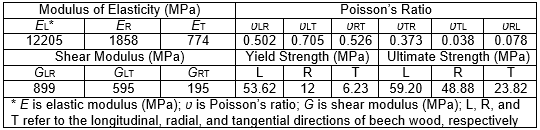Configurations of Specimen

Figure 1 shows the configurations of the M-T joint T-shaped specimens evaluated in this study. The dimensions of the leg were 150 mm long × 60 mm wide × 21 mm thick, and the sizes of the stretcher were 350 mm long × 60 mm wide × 21 mm thick. The tenon sizes varied in this study. According to the previous study (Wilczyński and Warmbier 2003), the tenon length was the greatest factor that affected the joint strength, which was followed by tenon width, and the effect of the tenon thickness was slight. Thus, in this study, the tenon length and tenon width were selected as two factors to evaluate the BMC of M-T joints to compare the finite element models proposed. The mortise and tenon were bonded by 65% solid content polyvinyl acetate (PVA) (Panda, Nanjing, China).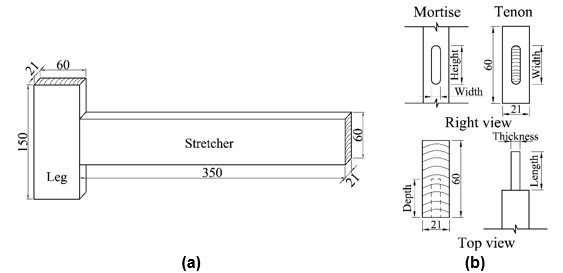Fig. 1. Dimensions of specimen: (a) T-shaped sample; (b) mortise-and-tenon joint

Finite Element Model

Figure 2 shows the finite element model of the M-T joint T-shaped samples established using finite element software (ABAQUS 6.14-1, Dassult, Providence, RI, USA) considering the orthotropic properties and large deformation of wood. The mechanical properties used in this model were determined from beech wood in previous studies (Table 1). Ductile damage was used as a stress criterion in the model. Local coordinates were used to define the grain orientations of the leg and the stretcher, i.e., x, y, and z corresponded to the longitudinal, radial, and tangential directions, respectively. In this study, three types of T-shaped joint finite element models were built and compared. The first model was a rigid model, in which the T-shaped joint was regarded as a whole rather than considering the mortise and tenon. The second model was also a rigid model; the T-shaped model was composed of a separate mortise and a separate tenon, but the mortise and tenon were connected via the tie method. The third model was a semi-rigid M-T joint T-shaped model. The interactions of the mortise and tenon were surface-to-surface contact. For the wide direction of the tenon, the penalty contact property was specified with a friction coefficient of 0.54 (Hu and Guan 2017b, Hu et al. 2018) to simulate the friction behavior between the mortise and tenon with a 0.2 mm interference fit. For the thick direction of the tenon, Cohesive Contact Property was applied to simulate the cohesive bonding behavior. The parameters needed in ABAQUS Knn, Kss and Ktt were 1.23, 3.49 and 2.45 N/mm, respectively. The loading conditions used were those of a previous study (Kasal et al. 2013), and a 30 mm displacement load was applied at the point 30 mm from the end of the stretcher to get the force (F) applied at the loading point (Fig. 2). The mesh of the model is also shown in Fig. 2, and the sizes of all elements were approximately 5 mm. For contact parts, the sizes of the elements were approximately 2 mm. The element type was C3D8, which is an 8-node linear brick element that was assigned to the T-shaped sample. The loading head was directed to R3D4, which is a 4-node 3-D bilinear rigid quadrilateral element.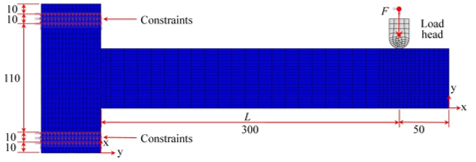Fig. 2. The finite element model used to simulate the bending moment capacity of the T-shaped mortise-and-tenon joint

Simulation Method

The joint type directly influences the strength of M-T joint T-shaped specimens. Furthermore, the method of establishing the finite element model of M-T joints affects the accuracy of simulations. In this study, three types of finite element M-T joint models were built and compared with the experimental results of a previous study (Kasal et al. 2013) to identify the best method to simulate the bending strengths of M-T joint T-shaped samples with the tenon sizes of 60 mm long × 30 mm wide × 7 mm thick. To further validate the accuracy of the finite element model, the effect of tenon length and tenon width on BMC and bending stiffness were investigated using the optimal model. When the effect of tenon length on BMC was studied, the tenon width and tenon thickness were kept as 30 mm and 7 mm, respectively, whereas the tenon length varied from 30 mm to 60 mm with 10 mm increments. When the effect of tenon width on BMC was studied, the tenon length and tenon thickness were kept constant at 40 mm and 7 mm, respectively, and the tenon widths were 20 mm, 30 mm, 40 mm, and 45 mm. Ten finite element models were established. The BMC and bending stiffness of the M-T joint T-shaped samples were calculated by Eq. 1 and Eq. 2, respectively,

F × (1)

= Δ/ Δ(2)

where M is bending moment capacity (Nm), F is ultimate force (N), L is length of moment arm (mm), K is stiffness (N/mm), ΔF is change of force in the linear portion of the bending load and deflection curve (mm), and Δd is the change in deflection corresponding to ΔF (mm).

RESULTS AND DISCUSSION

Comparisons of Different Finite Element Models

Figure 3 shows the stress distributions of three types of T-shaped finite element models containing two rigid joint models, which were the whole rigid model and the tie rigid model, and a semi-rigid model. For the whole rigid model of the T-shaped sample, the tenon was not separate until it reached the maximum bending load.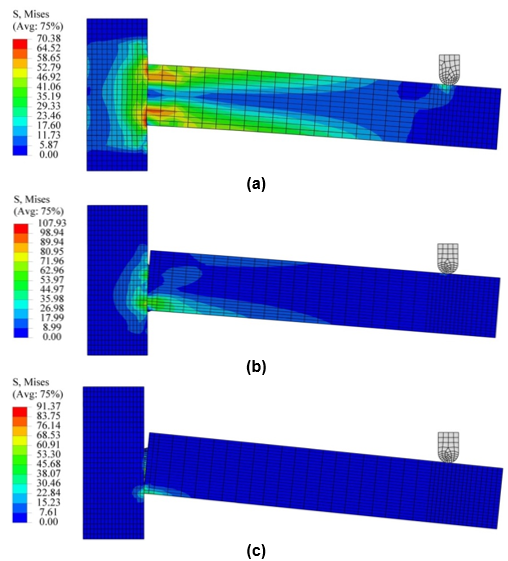Fig. 3. Stress distributions of the three T-shaped models subjected to bending load: (a) whole rigid model; (b) tie rigid model; and (c) semi-rigid model

For the tie rigid model, though a gap occurred between the joint, this gap was generated due to the deformation of mortise (Fig. 4a). For the semi-rigid joint, the tenon and mortise separated (Fig. 4b), and the tenon fractured when the bending force reached the peak value. Further comparison of the bending load and deflection curves of the three models revealed that the maximum bending load and stiffness of the rigid whole model were much bigger those of the tie rigid model and the semi-rigid model (Fig. 5). The BMCs of the whole rigid model, tie rigid model, and semi-rigid model were 937 N/m, 427 N/m, and 268 N/m, respectively. Compared with a previous study (Kasal et al. 2013), the BMC result for T-shaped samples of the same size was 250 N/m. All of the above comparisons indicated that the accuracy of the semi-rigid model was much higher than those of the whole rigid model and tie rigid model. Therefore, the semi-rigid finite element model was selected to numerically analyze the effects of tenon sizes on BMC and the bending stiffness of T-shaped joints.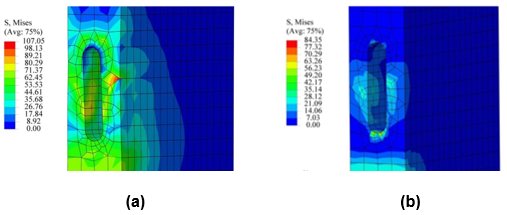Fig. 4. Stress distributions of mortise: (a) tie rigid model and (b) semi-rigid model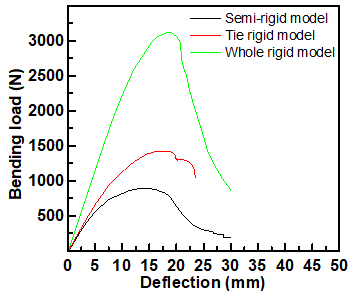Fig. 5. The bending load and deflection curves of T-shaped samples with different joint types

Effect of Tenon Size on Bending Strengths

Figure 6 shows a typical bending load and deflection curve of T-shaped joint with the tenon sizes of 60 mm long × 30 mm wide × 7 mm thick, which indicated the maximum bending load and stiffness.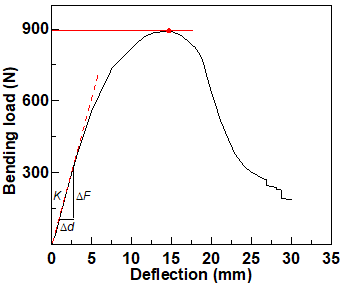Fig. 6. The typical bending load and deflection curve of the mortise-and-tenon joint

Figure 7 shows the stress distributions of the semi-rigid model of T-shaped joint with the tenon sizes of 60 mm long × 30 mm wide × 7 mm thick, which presented four typical states during the loading process from the initial load to the failure load with the tenon fracture.